This article is mainly to summarize and refine the content of “100 Go Mistakes How to Avoid Them”, both veterans and novices can actually read it, many problems are easy to be ignored. The author of this book is also very difficult, taking into account so many problems that arise when using Go.

 `````` 1 2 3 4 5 6 7 8 9 10 11 12 13 14 `````` `````` var client *http.Client if tracing { client, err := createClientWithTracing() if err != nil { return err } log.Println(client) } else { client, err := createDefaultClient() if err != nil { return err } log.Println(client) } ``````

In the above code, a client variable is declared, and then tracing is used to control the initialization of the variable, probably because err is not declared, and `:=` is used for initialization, which causes the outer client variable to always be nil. this example is actually very easy to happen in our actual development, and requires particular attention.

If it’s because err is not initialized, we can do this when we initialize it.

 `````` 1 2 3 4 5 6 7 8 9 10 `````` `````` var client *http.Client var err error if tracing { client, err = createClientWithTracing() } else { ... } if err != nil { // Preventing duplicate codes return err } ``````

Or simply change the variable name for the inner variable declaration, so that it is less prone to errors.

We can also use a tool to analyze the code for shadowing by first installing the following tool:

 ``````1 `````` ``````go install golang.org/x/tools/go/analysis/passes/shadow/cmd/shadow ``````

 ``````1 2 3 4 `````` `````` go vet -vettool=C:\Users\luozhiyun\go\bin\shadow.exe .\main.go # command-line-arguments .\main.go:15:3: declaration of "client" shadows declaration at line 13 .\main.go:21:3: declaration of "client" shadows declaration at line 13 ``````

## Use the init function with care

There are a few things to keep in mind before using the init function:

### The init function will be executed after global variables

The init function is not the first to be executed. If const or global variables are declared, the init function will be executed after them:

 `````` 1 2 3 4 5 6 7 8 9 10 11 12 13 14 15 16 17 18 19 20 21 `````` ``````package main import "fmt" var a = func() int { fmt.Println("a") return 0 }() func init() { fmt.Println("init") } func main() { fmt.Println("main") } // output a init main ``````

### init initialization is executed in the order of the resolved dependencies

For example, if the main package has an init function that depends on the redis package, and the main function executes the Store function of the redis package, and it happens that the redis package also has an init function, then the order of execution will be as follows.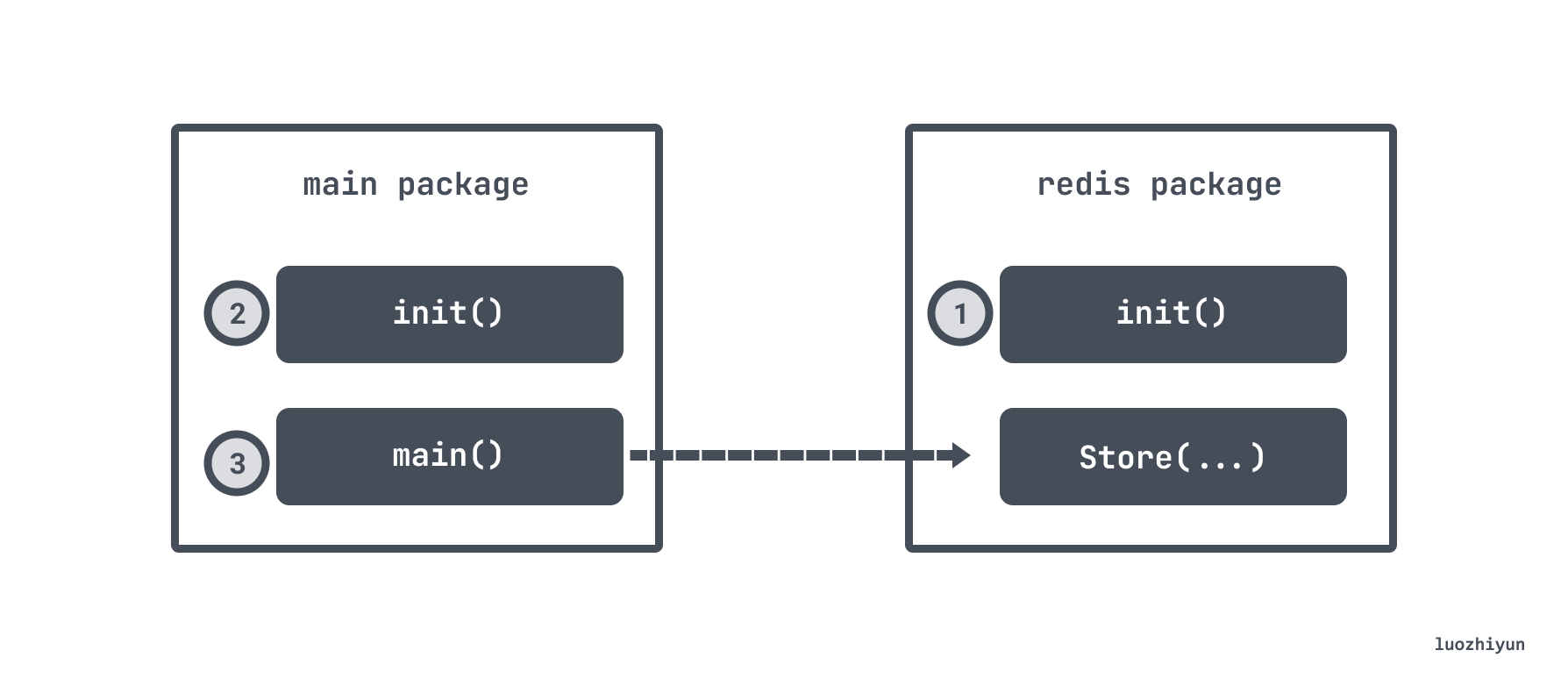In another case, if it is introduced using `"import _ foo"`, the init function in the foo package is also called first.

### Disrupting unit tests

For example, if we initialize a global variable in the init function, but it is not needed in the unit test, it actually increases the complexity of the unit test, e.g:

 ``````1 2 3 4 5 6 7 8 9 `````` ``````var db *sql.DB func init(){ dataSourceName := os.Getenv("MYSQL_DATA_SOURCE_NAME") d, err := sql.Open("mysql", dataSourceName) if err != nil { log.Panic(err) } db = d } ``````

In the above example the init function initializes a db global variable. Then a unit test will also initialize such a variable, but many unit tests are actually quite simple and do not rely on this.

embed types refer to the anonymous fields we define inside the struct, e.g:

 ``````1 2 3 4 5 6 `````` ``````type Foo struct { Bar } type Bar struct { Baz int } ``````

In the above example, we can access the member variables directly through `Foo.Baz`, and of course through `Foo.Bar.Baz`.

This can increase our ease of use in many cases, if we don’t use embed types then it may require a lot of code, as follows:

 `````` 1 2 3 4 5 6 7 8 9 10 11 12 13 14 15 16 17 `````` ``````type Logger struct { writeCloser io.WriteCloser } func (l Logger) Write(p []byte) (int, error) { return l.writeCloser.Write(p) } func (l Logger) Close() error { return l.writeCloser.Close() } func main() { l := Logger{writeCloser: os.Stdout} _, _ = l.Write([]byte("foo")) _ = l.Close() } ``````

If we use embed types our code can be very clean.

 ``````1 2 3 4 5 6 7 8 9 `````` ``````type Logger struct { io.WriteCloser } func main() { l := Logger{WriteCloser: os.Stdout} _, _ = l.Write([]byte("foo")) _ = l.Close() } ``````

But it also has the disadvantage that some fields we don’t want to export, but embed types may bring us out, e.g:

 ``````1 2 3 4 5 6 7 8 `````` ``````type InMem struct { sync.Mutex m map[string]int } func New() *InMem { return &InMem{m: make(map[string]int)} } ``````

Mutexes generally don’t want to export, they just want to be used in InMem’s own functions, e.g:

 ``````1 2 3 4 5 6 `````` ``````func (i *InMem) Get(key string) (int, bool) { i.Lock() v, contains := i.m[key] i.Unlock() return v, contains } ``````

But writing it this way allows all variables of type InMem to use its Lock method.

 ``````1 2 `````` ``````m := inmem.New() m.Lock() // ?? ``````

## Functional Options Pattern passing parameters

This approach has been seen in use in many Go open source libraries, such as zap, GRPC, etc.

It is often used when you need to pass and initialize a list of check parameters, for example, we now need to initialize an HTTP server, which may contain information such as port, timeout, etc., but the list of parameters is large and cannot be written directly on the function, and we want to meet the requirements of flexible configuration, after all, not every server requires many parameters. Then we can:

• set up a non-exportable struct called options to hold the configuration parameters;
• create a type `type Option func(options *options) error`, and use this type as the return value;

For example, if we want to set a port parameter inside the HTTP server, we can declare a WithPort function that returns a closure of type Option, which will be populated with the port of options when the closure is executed:

 `````` 1 2 3 4 5 6 7 8 9 10 11 12 13 14 15 16 `````` ``````type options struct { port *int } type Option func(options *options) error func WithPort(port int) Option { // All the type checking, assignment, initialization and so on can be done in this closure return func(options *options) error { if port < 0 { return errors.New("port should be positive") } options.port = &port return nil } } ``````

Suppose we now have a set of Option functions like this, which can be populated with timeout, etc., in addition to the port above. Then we can use NewServer to create our server.

 `````` 1 2 3 4 5 6 7 8 9 10 11 12 13 14 15 16 17 18 19 20 21 22 23 24 25 `````` ``````func NewServer(addr string, opts ...Option) (*http.Server, error) { var options options // Iterate through all Option for _, opt := range opts { //Execution of closures err := opt(&options) if err != nil { return nil, err } } //Next, we can fill in our business logic, such as setting the default port here, etc. var port int if options.port == nil { port = defaultHTTPPort } else { if *options.port == 0 { port = randomPort() } else { port = *options.port } } // ... } ``````

Initialize server:

 ``````1 2 3 `````` ``````server, err := httplib.NewServer("localhost", httplib.WithPort(8080), httplib.WithTimeout(time.Second)) ``````

It’s more flexible to write it this way, so if we just want to generate a simple server, our code can become very simple.

 ``````1 `````` ``````server, err := httplib.NewServer("localhost") ``````

## Beware of octal integers

For example, the following example:

 ``````1 2 `````` `````` sum := 100 + 010 fmt.Println(sum) ``````

You think you’re outputting 110, but you’re actually outputting 108, because in Go integers starting with 0 represent octal.

It is often used to handle Linux permissions-related code, such as the following to open a file:

 ``````1 `````` `````` file, err := os.OpenFile("foo", os.O_RDONLY, 0644) ``````

So for readability, it is better to use the “0o” representation when we use octal, for example, the above code can be represented as

 ``````1 `````` `````` file, err := os.OpenFile("foo", os.O_RDONLY, 0o644) ``````

## The precision of float

In Go, floats are represented by scientific notation, just like other languages. floats are stored in three parts

1. Sign: 0 means positive, 1 means negative
2. Exponent: used to store exponential data in scientific notation, and the use of shift storage
3. Mantissa: the trailing part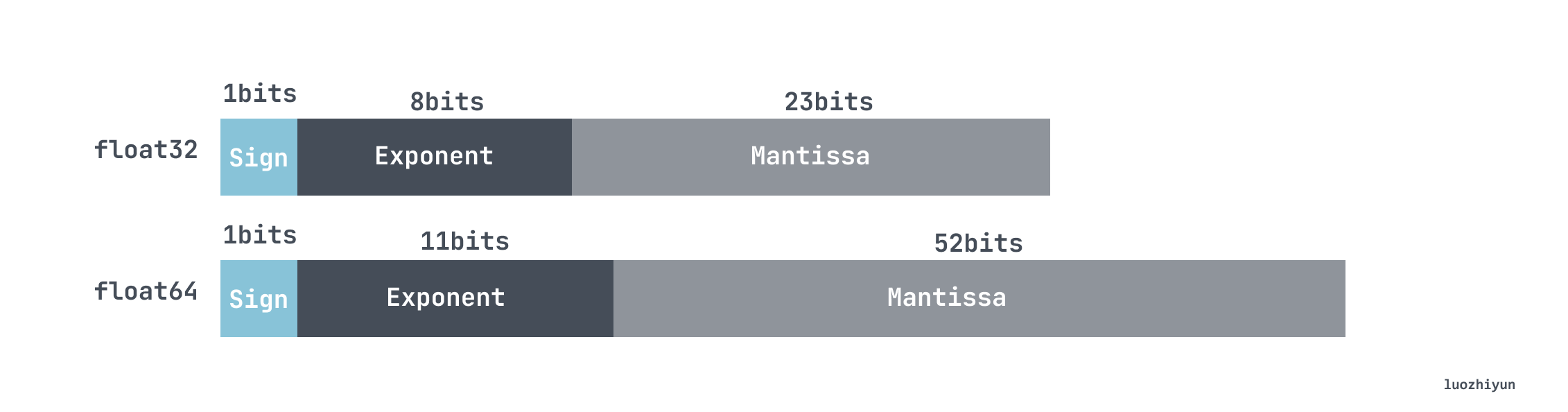I’m not going to show the rules here, so you can explore them yourself if you’re interested. I’ll talk about what problems this counting method has in Go.

 `````` 1 2 3 4 5 6 7 8 9 10 11 12 13 14 15 `````` ``````func f1(n int) float64 { result := 10_000. for i := 0; i < n; i++ { result += 1.0001 } return result } func f2(n int) float64 { result := 0. for i := 0; i < n; i++ { result += 1.0001 } return result + 10_000. } ``````

In this code above, we simply do some addition.

n Exact result `f1` `f2`
10 10010.001 10010.000999999993 10010.001
1k 11000.1 11000.099999999293 11000.099999999982
1m 1.0101e+06 1.0100999999761417e+06 1.0100999999766762e+06

We can see that the larger n is, the larger the error is, and the error of f2 is smaller than f1.

For multiplication we can do the following experiment:

 ``````1 2 3 4 5 6 `````` ``````a := 100000.001 b := 1.0001 c := 1.0002 fmt.Println(a * (b + c)) fmt.Println(a*b + a*c) ``````

Output:

 ``````1 2 `````` ``````200030.00200030004 200030.0020003 ``````

The correct output would be 200030.0020003, so they are actually both in error, but you can see that the precision loss is smaller when multiplying first and then adding.

If you want to calculate floating point accurately, you can try the `"github.com/shopspring/decimal"` library and switch to this library and let’s calculate:

 ``````1 2 3 4 5 `````` ``````a := decimal.NewFromFloat(100000.001) b := decimal.NewFromFloat(1.0001) c := decimal.NewFromFloat(1.0002) fmt.Println(a.Mul(b.Add(c))) //200030.0020003 ``````

### Distinguish between length and capacity of a slice

First let’s initialize a slice with length and capacity:

 ``````1 `````` ``````s := make([]int, 3, 6) ``````

In the make function, capacity is an optional parameter. The above code creates a slice with length 3 and capacity 6, so the underlying data structure looks like this: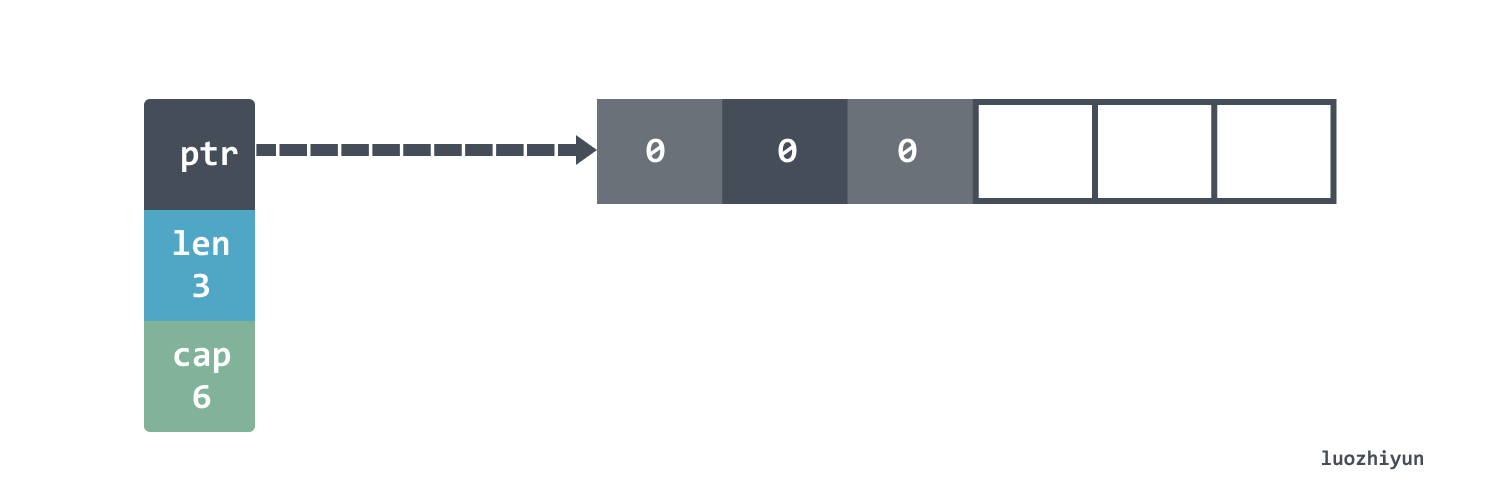The bottom of the slice actually points to an array. Of course, since our length is 3, setting `s = 0` in this way would be panic. You need to use `append` to add new elements.

 ``````1 `````` ``````panic: runtime error: index out of range  with length 3 ``````

When appned exceeds the cap size, the slice will automatically expand for us, doubling each time the number of elements is less than 1024, and 25% each time the number of elements exceeds 1024.

Sometimes we use the `:` operator to create a new slice from another slice:

 ``````1 2 `````` ``````s1 := make([]int, 3, 6) s2 := s1[1:3] ``````

In fact, the two slices still point to the same underlying array, constructed as follows: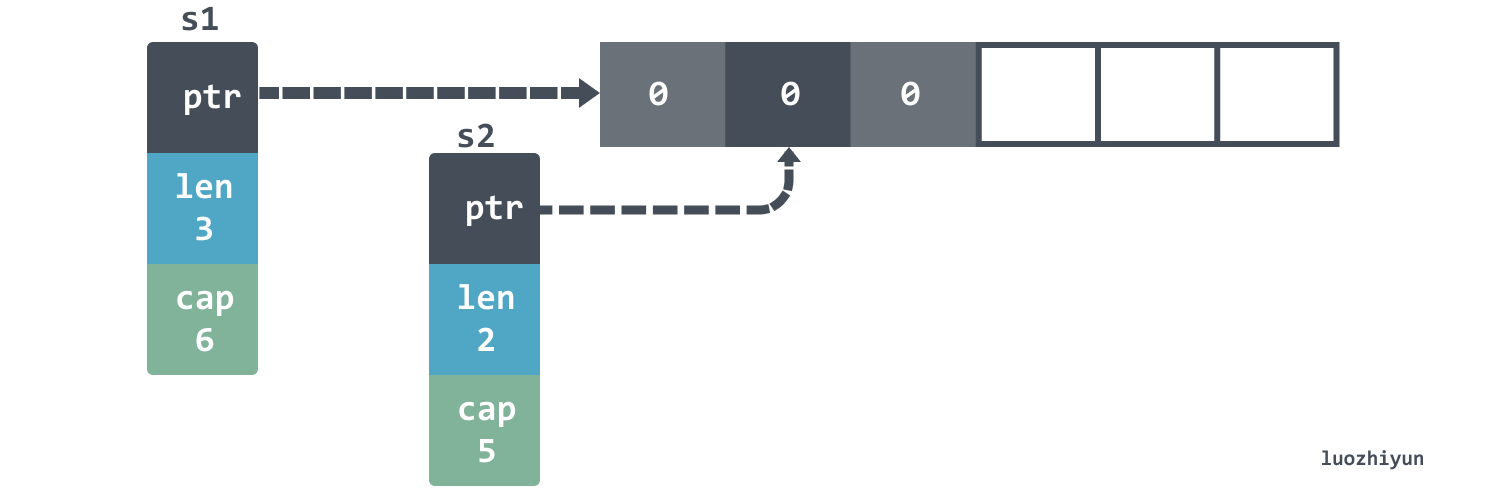Since it points to the same array, then when we change the first slot, say `s1=2`, the data of both slice will actually change.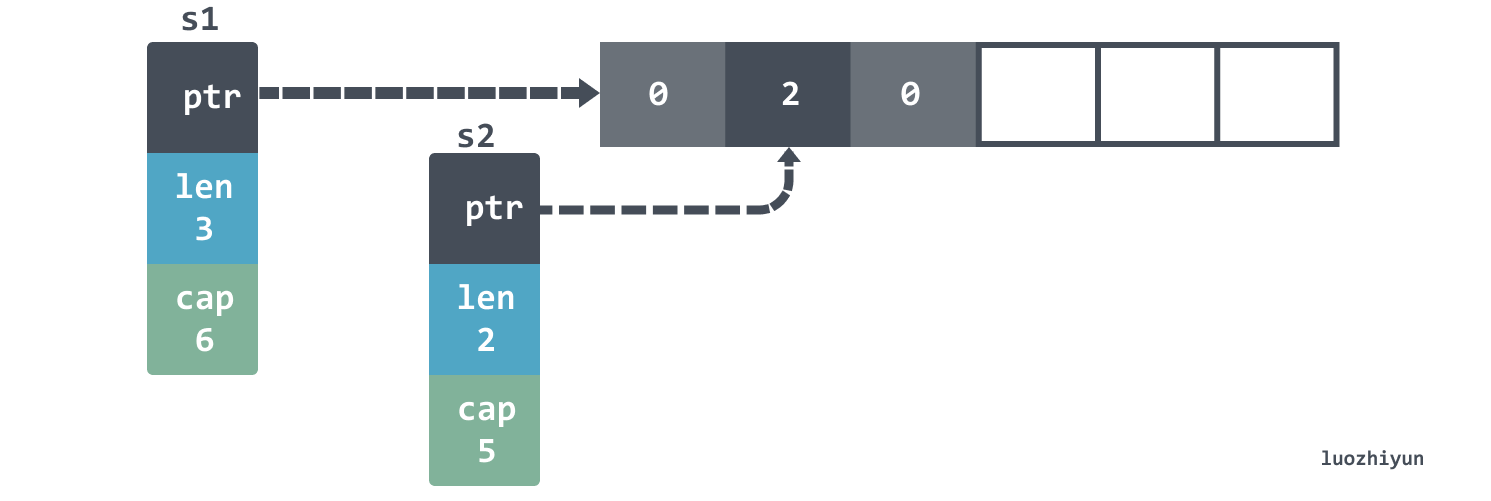But the situation is different when we use append.

 ``````1 2 3 4 `````` ``````s2 = append(s2, 3) fmt.Println(s1) // [0 2 0] fmt.Println(s2) // [2 0 3] ``````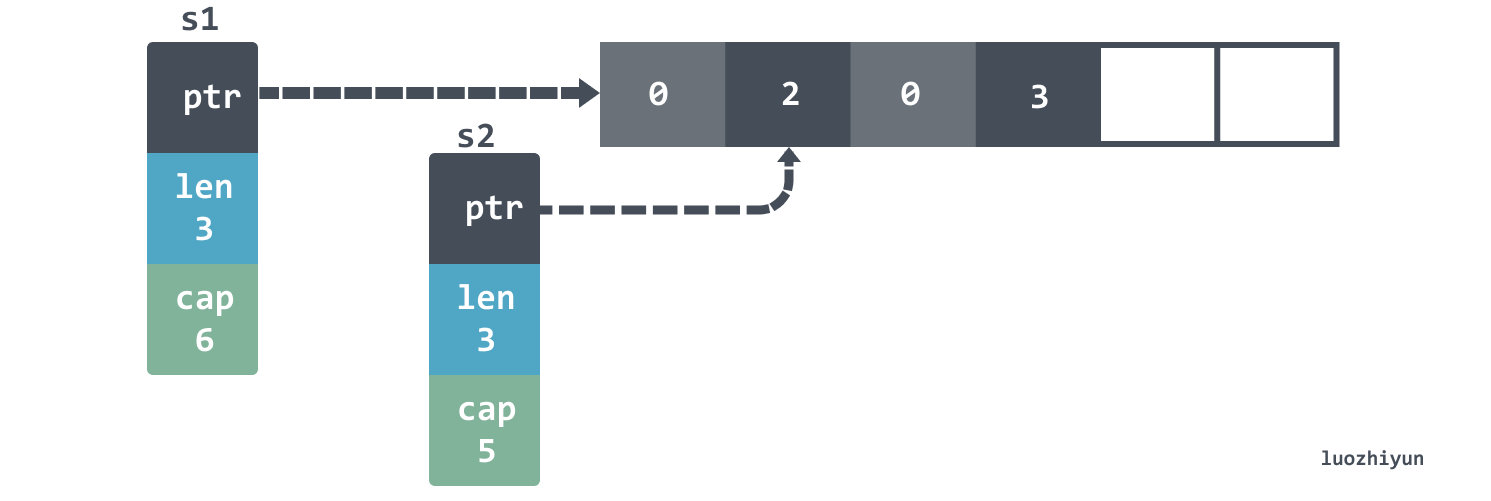The len of s1 is not changed, so we still see 3 elements.

Another interesting detail is that if you then append s1 then the fourth element will be overwritten: the

 ``````1 2 3 `````` `````` s1 = append(s1, 4) fmt.Println(s1) // [0 2 0 4] fmt.Println(s2) // [2 0 4] ``````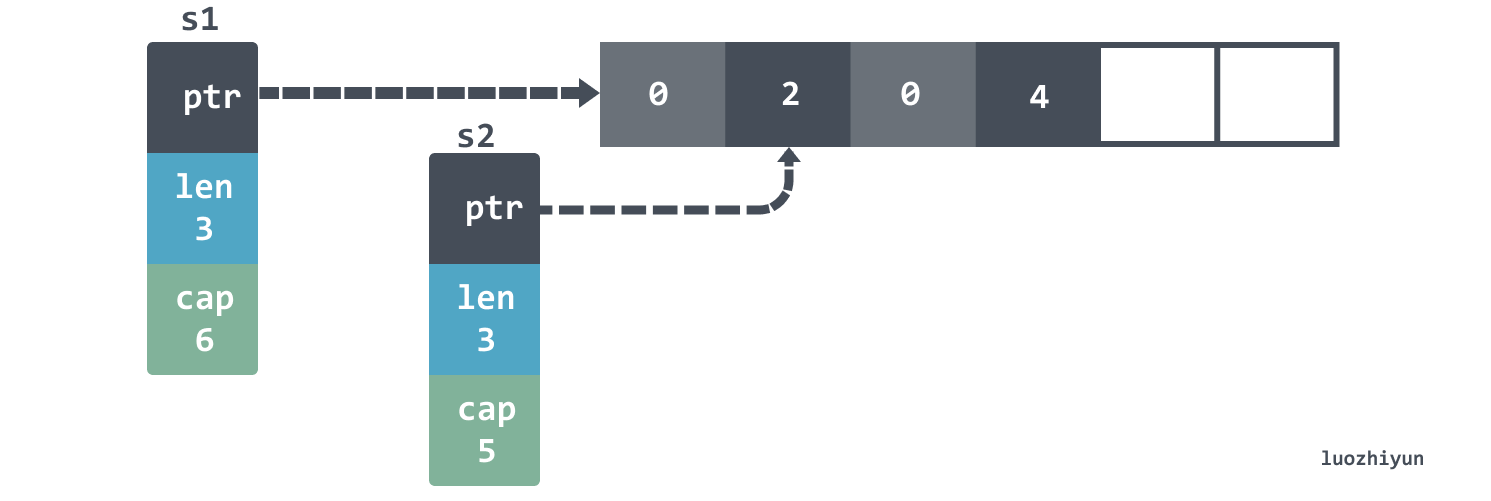We continue to append s2 until s2 is expanded, at which point we find that s2 is not actually pointing to the same array as s1.

 ``````1 2 3 `````` ``````s2 = append(s2, 5, 6, 7) fmt.Println(s1) //[0 2 0 4] fmt.Println(s2) //[2 0 4 5 6 7] ``````

In addition to the above case, there is another case where append can have an unexpected effect.

 ``````1 2 3 `````` ``````s1 := []int{1, 2, 3} s2 := s1[1:2] s3 := append(s2, 10) ``````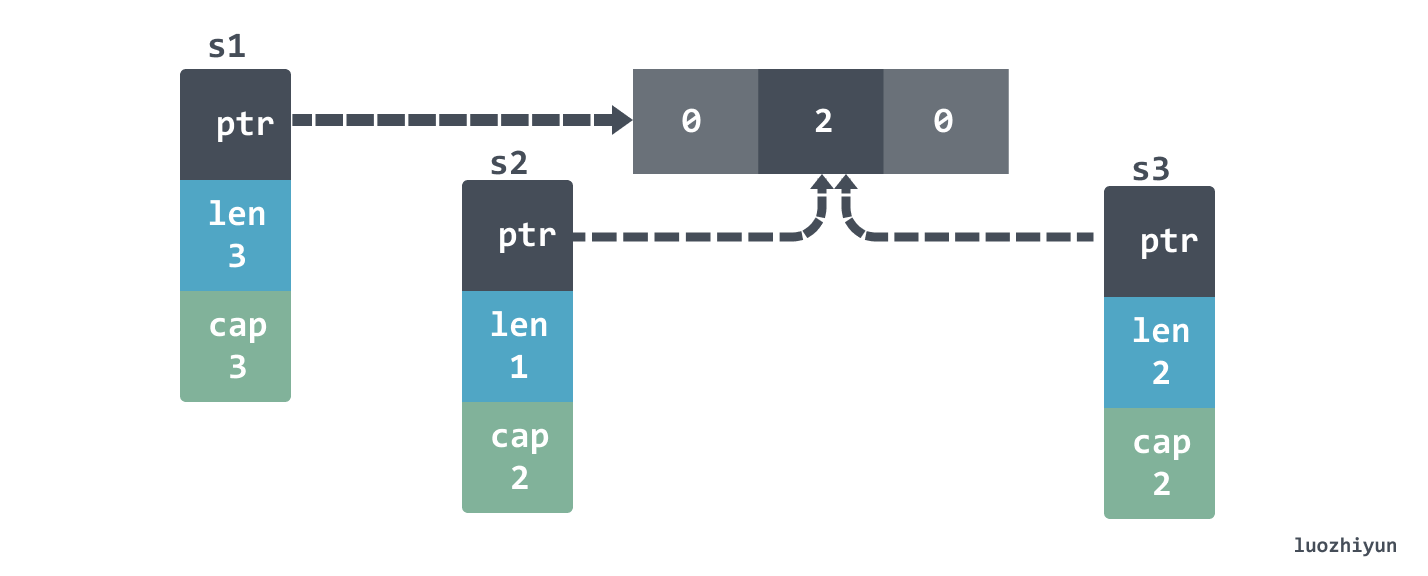If print they should look like this:

 ``````1 `````` ``````s1=[1 2 10], s2=, s3=[2 10] ``````

### slice initialization

There are actually many ways to initialize a slice:

 `````` 1 2 3 4 5 6 7 8 9 10 11 12 13 14 15 16 17 `````` ``````func main() { var s []string log(1, s) s = []string(nil) log(2, s) s = []string{} log(3, s) s = make([]string, 0) log(4, s) } func log(i int, s []string) { fmt.Printf("%d: empty=%t\tnil=%t\n", i, len(s) == 0, s == nil) } ``````

Output:

 ``````1 2 3 4 `````` ``````1: empty=true nil=true 2: empty=true nil=true 3: empty=true nil=false 4: empty=true nil=false ``````

The first two ways create a nil slice, the last two initialize it and the size of these slice is 0.

For the `var s []string` approach, the advantage is that no memory allocation is needed. For example, the following scenario may save a memory allocation:

 `````` 1 2 3 4 5 6 7 8 9 10 `````` ``````func f() []string { var s []string if foo() { s = append(s, "foo") } if bar() { s = append(s, "bar") } return s } ``````

For the `s := []string{}` approach, which is better suited for initializing a slice with known elements:

 ``````1 `````` ``````s := []string{"foo", "bar", "baz"} ``````

If we don’t have this need, it’s better to use `var s []string`, since we add elements by append anyway, and `var s []string` saves one memory allocation.

If we initialize an empty slice, it is better to use `len(xxx) == 0` to determine whether the slice is empty or not, if we use nil to determine it may always be non-empty, because for `s := []string{}` and `s = make([]string, 0)` both initializations are non-nil.

For `[]string(nil)` this initialization is rarely used, a more convenient scenario is to use it for copy of slice:

 ``````1 2 `````` ``````src := []int{0, 1, 2} dst := append([]int(nil), src...) ``````

For make, it can initialize the length and capacity of the slice, if we can determine how many elements will be stored inside the slice, from the performance point of view, it is best to use make to initialize the good, because for an empty slice append elements into each time to reach the threshold need to expand the capacity, the following is the filling of 1 million elements benchmark.

 ``````1 2 3 `````` ``````BenchmarkConvert_EmptySlice-4 22 49739882 ns/op BenchmarkConvert_GivenCapacity-4 86 13438544 ns/op BenchmarkConvert_GivenLength-4 91 12800411 ns/op ``````

As you can see, if we fill the slice size in advance, the performance is four times higher than an empty slice, because there is less overhead of copying elements and reapplying new arrays during expansion.

### copy slice

 ``````1 2 3 4 `````` ``````src := []int{0, 1, 2} var dst []int copy(dst, src) fmt.Println(dst) // [] ``````

When using the copy function to copy slice, it should be noted that the above case will actually fail to copy, because for slice the available data is controlled by length, copy does not copy this field, to copy we can do the following:

 ``````1 2 3 4 `````` ``````src := []int{0, 1, 2} dst := make([]int, len(src)) copy(dst, src) fmt.Println(dst) //[0 1 2] ``````

In addition to this it is also possible to use the above mentioned:

 ``````1 2 `````` ``````src := []int{0, 1, 2} dst := append([]int(nil), src...) ``````

### slice capacity memory release problem

 `````` 1 2 3 4 5 6 7 8 9 10 11 12 13 14 15 16 17 18 19 20 21 22 23 24 `````` ``````type Foo struct { v []byte } func keepFirstTwoElementsOnly(foos []Foo) []Foo { return foos[:2] } func main() { foos := make([]Foo, 1_000) printAlloc() for i := 0; i < len(foos); i++ { foos[i] = Foo{ v: make([]byte, 1024*1024), } } printAlloc() two := keepFirstTwoElementsOnly(foos) runtime.GC() printAlloc() runtime.KeepAlive(two) } ``````

The printAlloc function is used in the above example to print the memory footprint:

 ``````1 2 3 4 5 `````` ``````func printAlloc() { var m runtime.MemStats runtime.ReadMemStats(&m) fmt.Printf("%d KB\n", m.Alloc/1024) } ``````

The above foos initializes a 1000 capacity slice, in which each Foo struct holds a 1M memory slice, and then returns the Foo slice holding the first two elements by keepFirstTwoElementsOnly, our idea is to manually execute GC and then the other 998 Foo will be destroyed by GC, but the output The result is as follows:

 ``````1 2 3 `````` ``````387 KB 1024315 KB 1024319 KB ``````

Actually, it doesn’t, because the slice returned by keepFirstTwoElementsOnly actually holds the same array as foos does.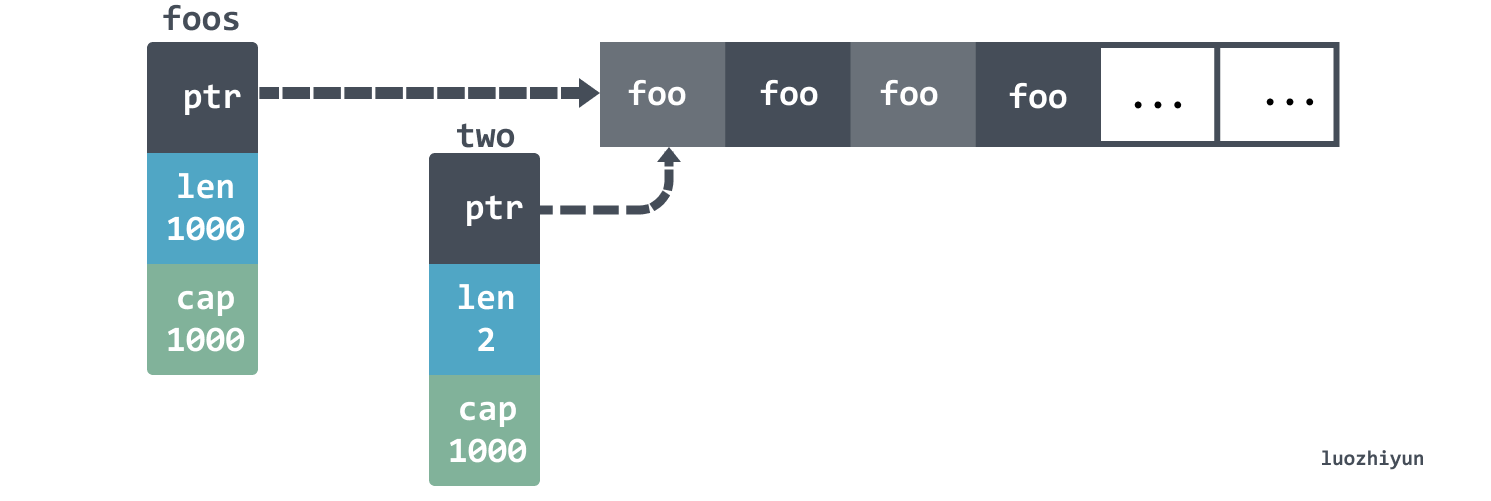So if we really want to return only the first 2 elements of a slice, we should do it like this:

 ``````1 2 3 4 5 `````` ``````func keepFirstTwoElementsOnly(foos []Foo) []Foo { res := make([]Foo, 2) copy(res, foos) return res } ``````

However, the above method initializes a new slice and then copies the two elements over. You can do this if you don’t want to make redundant allocations:

 ``````1 2 3 4 5 6 `````` ``````func keepFirstTwoElementsOnly(foos []Foo) []Foo { for i := 2; i < len(foos); i++ { foos[i].v = nil } return foos[:2] } ``````

## Note the range

### Problems with copy

When using range, if we directly modify the data it returns, it will not work, because the data at the time of iteration is not the original data:

 `````` 1 2 3 4 5 6 7 8 9 10 11 12 `````` ``````type account struct { balance float32 } accounts := []account{ {balance: 100.}, {balance: 200.}, {balance: 300.}, } for _, a := range accounts { a.balance += 1000 } ``````

If this is done as above, the output accounts are:

 ``````1 `````` ``````[{100} {200} {300}] ``````

So we want to change the data in the range by doing this:

 ``````1 2 3 `````` ``````for i := range accounts { accounts[i].balance += 1000 } ``````

slice is also copied during range.

 ``````1 2 3 4 `````` ``````s := []int{0, 1, 2} for range s { s = append(s, 10) } ``````

This code will be copied during range, so it will only call append three times and then stop.

### Problems with pointer

 `````` 1 2 3 4 5 6 7 8 9 10 11 12 13 14 `````` ``````type Customer struct { ID string Balance float64 } test := []Customer{ {ID: "1", Balance: 10}, {ID: "2", Balance: -10}, {ID: "3", Balance: 0}, } for _, customer := range test { fmt.Printf("%p\n", &customer) //When we want to get this pointer } ``````

Output:

 ``````1 2 3 `````` ``````0x1400000e240 0x1400000e240 0x1400000e240 ``````

This is because the iterator puts all the data into the 0x1400000e240 space, so it is actually taking the iterator’s pointer.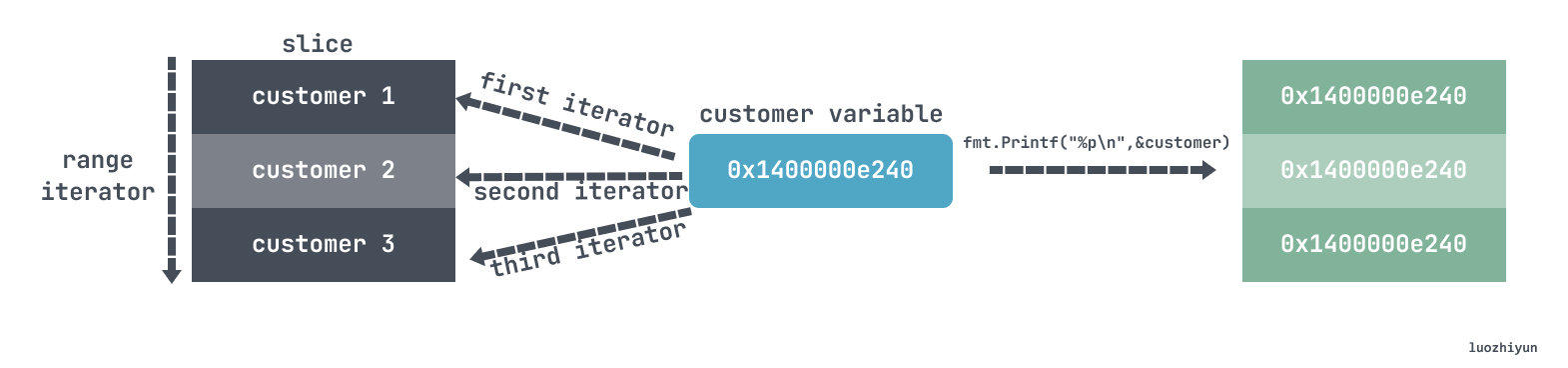The correct traversal to fetch a pointer should look like this:

 ``````1 2 3 4 `````` `````` for _, customer := range test { current := customer fmt.Printf("%p\n", ¤t) } ``````

## Note the break scope

Let’s say:

 ``````1 2 3 4 5 6 7 8 9 `````` ``````for i := 0; i < 5; i++ { fmt.Printf("%d ", i) switch i { default: case 2: break } } ``````

The above code is meant to break and stop the traversal, but in fact it just breaks the switch scope, and print still prints: 0, 1, 2, 3, 4.

The correct way to do this would be to break by labeling:

 ``````1 2 3 4 5 6 7 8 9 `````` ``````loop: for i := 0; i < 5; i++ { fmt.Printf("%d ", i) switch i { default: case 2: break loop } } ``````

Sometimes we don’t notice our wrong usage, such as the following:

 ``````1 2 3 4 5 6 7 8 `````` `````` for { select { case <-ch: // Do something case <-ctx.Done(): break } } ``````

The way it is written above will cause only select to exit, and not terminate the for loop. The correct way to write it would be like this:

 ``````1 2 3 4 5 6 7 8 9 `````` `````` loop: for { select { case <-ch: // Do something case <-ctx.Done(): break loop } } ``````

## defer

### Pay attention to the timing of defer calls

Sometimes we use defer to close some resources like the following:

 `````` 1 2 3 4 5 6 7 8 9 10 11 12 13 `````` ``````func readFiles(ch <-chan string) error { for path := range ch { file, err := os.Open(path) if err != nil { return err } defer file.Close() // Do something with file } return nil } ``````

Because defer will be called at the end of the method, but if the readFiles function above never returns, then defer will never be called, resulting in a memory leak. Also, defer is written inside a for loop and the compiler cannot optimize it, which affects code execution performance.

To avoid this, we can wrap it.

 `````` 1 2 3 4 5 6 7 8 9 10 11 12 13 14 15 16 17 18 19 20 `````` ``````func readFiles(ch <-chan string) error { for path := range ch { if err := readFile(path); err != nil { return err } } return nil } func readFile(path string) error { file, err := os.Open(path) if err != nil { return err } defer file.Close() // Do something with file return nil } ``````

### Note the arguments to defer

The defer declaration will first calculate the value of the argument.

 `````` 1 2 3 4 5 6 7 8 9 10 `````` ``````func a() { i := 0 defer notice(i) // 0 i++ return } func notice(i int) { fmt.Println(i) } ``````

In this example, the variable i is determined when `defer` is called, not when `defer` is executed, so the output of the above statement is 0.

So if we want to get the real value of this variable, we should use the reference:

 ``````1 2 3 4 5 6 `````` ``````func a() { i := 0 defer notice(&i) // 0 i++ return } ``````

### closures under defer

 `````` 1 2 3 4 5 6 7 8 9 10 11 12 `````` ``````func a() int { i := 0 defer func() { fmt.Println(i + 1) //12 }() i++ return i+10 } func TestA(t *testing.T) { fmt.Println(a()) //11 } ``````

If we switch to closures, we can actually read the real value of variable i in the closure because it is passed through a pointer. But in the example above, the a function returns 11 because the order of execution is

 ``````1 `````` ``````Calculate (i+10) first -> (call defer) -> (return) ``````

### Problems with iteration

In Go, a string is a basic type, which by default is a sequence of characters encoded in utf8, taking up 1 byte when the character is ASCII, and 2-4 bytes for other characters as needed, e.g. Chinese encoding usually takes 3 bytes.

Then we may have unexpected problems when doing string iterations:

 ``````1 2 3 4 5 `````` `````` s := "hêllo" for i := range s { fmt.Printf("position %d: %c\n", i, s[i]) } fmt.Printf("len=%d\n", len(s)) ``````

Output:

 ``````1 2 3 4 5 6 `````` ``````position 0: h position 1: Ã position 3: l position 4: l position 5: o len=6 ``````

The above output shows that the second character is Ã, not ê, and the output of position 2 “disappears”, because ê actually occupies 2 bytes in utf8:

s h ê l l o
[]byte(s) 68 c3 aa 6c 6c 6f

So when we iterate s equals c3, the byte equals Ã, the utf8 value, so the output is `hÃllo` instead of `hÃllo`.

Then, based on the above analysis, we know that when iterating over the characters we can’t just get a single byte, but should use the value returned by range:

 ``````1 2 3 4 `````` `````` s := "hêllo" for i, v := range s { fmt.Printf("position %d: %c\n", i, v) } ``````

Or we can convert the string to a rune array, which stands for `Unicode` code in go, and use it to output a single character.

 ``````1 2 3 4 5 `````` `````` s := "hêllo" runes := []rune(s) for i, _ := range runes { fmt.Printf("position %d: %c\n", i, runes[i]) } ``````

Output:

 ``````1 2 3 4 5 `````` ``````position 0: h position 1: ê position 2: l position 3: l position 4: o ``````

### Problems caused by truncation

As we mentioned above when we talked about slice, when using the `:` operator to truncate slice, the underlying array is actually pointing to the same one, and you need to pay attention to this problem in string as well, such as the following:

 ``````1 2 3 4 5 6 7 8 `````` ``````func (s store) handleLog(log string) error { if len(log) < 36 { return errors.New("log is not correctly formatted") } uuid := log[:36] s.store(uuid) // Do something } ``````

This code uses the `:` operator for truncation, but if the log object is large, like the store method above that keeps the uuid in memory, it may cause the underlying array to remain unfree, thus causing a memory leak.

To solve this problem, we can make a copy before processing:

 ``````1 2 3 4 5 6 7 8 `````` ``````func (s store) handleLog(log string) error { if len(log) < 36 { return errors.New("log is not correctly formatted") } uuid := strings.Clone(log[:36]) // copy一份 s.store(uuid) // Do something } ``````

## interface type returns non-nil problem

Suppose we want to inherit the error interface to implement a MultiError of our own:

 `````` 1 2 3 4 5 6 7 8 9 10 11 `````` ``````type MultiError struct { errs []string } func (m *MultiError) Add(err error) { m.errs = append(m.errs, err.Error()) } func (m *MultiError) Error() string { return strings.Join(m.errs, ";") } ``````

Then return error when used, and want to determine if there is an error by whether error is nil or not:

 `````` 1 2 3 4 5 6 7 8 9 10 11 12 13 14 15 16 17 18 19 20 21 `````` ``````func Validate(age int, name string) error { var m *MultiError if age < 0 { m = &MultiError{} m.Add(errors.New("age is negative")) } if name == "" { if m == nil { m = &MultiError{} } m.Add(errors.New("name is nil")) } return m } func Test(t *testing.T) { if err := Validate(10, "a"); err != nil { t.Errorf("invalid") } } ``````

In fact, the err returned by Validate will always be non-nil, which means that the above code will only output `invalid` .

 ``````1 `````` ``````invalid ``````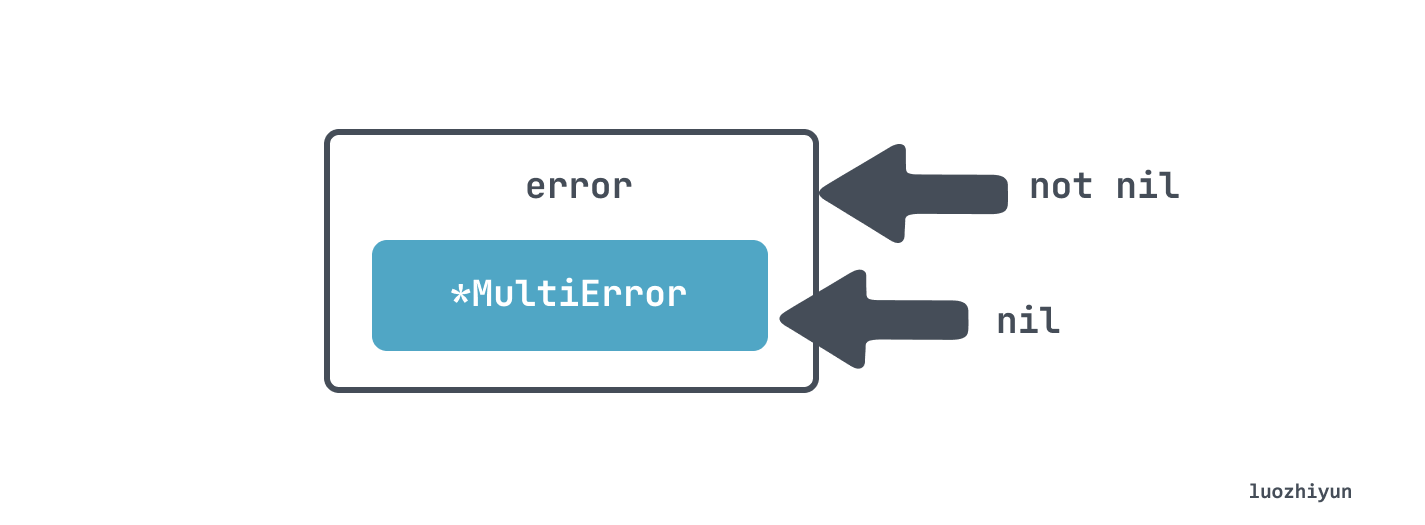## Error

### error wrap

For the return of err we can generally handle it like this:

 ``````1 2 3 4 `````` `````` err:= xxx() if err != nil { return err } ``````

But this simply throws out the original error, and there is no way to know the context of the program being processed, so we might customize the error structure to inherit from the error interface:

 ``````1 2 3 4 `````` `````` err:= xxx() if err != nil { return XXError{Err: err} } ``````

Then we add all the context information to XXError, but although this can add some context information, it becomes troublesome to create a specific type of error class each time, so after 1.13, we can use `%w` to wrap.

 ``````1 2 3 `````` `````` if err != nil { return fmt.Errorf("xxx failed: %w", err) } ``````

Of course, in addition to the above approach, we can also directly `%v` format our error messages directly.

 ``````1 2 3 `````` `````` if err != nil { return fmt.Errorf("xxx failed: %v", err) } ``````

The disadvantage of this is that we lose the type information of the err, but if we don’t need the type information and just want to throw some logs upwards, it doesn’t matter.

### error Is & As

Because our error can be wrap several layers, so using `==` may not be able to determine if our error is the specific error we want, so we can use `errors.Is`.

 `````` 1 2 3 4 5 6 7 8 9 10 11 12 `````` ``````var BaseErr = errors.New("base error") func main() { err1 := fmt.Errorf("wrap base: %w", BaseErr) err2 := fmt.Errorf("wrap err1: %w", err1) println(err2 == BaseErr) if !errors.Is(err2, BaseErr) { panic("err2 is not BaseErr") } println("err2 is BaseErr") } ``````

Output:

 ``````1 2 `````` ``````false err2 is BaseErr ``````

Above, we can tell that err2 contains a BaseErr error by using `errors.Is`. `errors.Is` recursively calls the `Unwrap` method to unwrap the errors, and then uses `==` one by one to determine if they are equal to the specified type of error.

`errors.As` is mainly used for type determination, for the same reason as above, after the error is wrap we can’t determine it directly by `err(type)`, `errors.As` will unwrap it with `Unwrap` method and then determine the type one by one. The way it is used is as follows:

 `````` 1 2 3 4 5 6 7 8 9 10 11 12 13 14 15 16 17 18 19 `````` ``````type TypicalErr struct { e string } func (t TypicalErr) Error() string { return t.e } func main() { err := TypicalErr{"typical error"} err1 := fmt.Errorf("wrap err: %w", err) err2 := fmt.Errorf("wrap err1: %w", err1) var e TypicalErr if !errors.As(err2, &e) { panic("TypicalErr is not on the chain of err2") } println("TypicalErr is on the chain of err2") println(err == e) } ``````

Output:

 ``````1 2 `````` ``````TypicalErr is on the chain of err2 true ``````

### Handling error in defer

For example, the following code, if we return an error when calling Close, is not handled.

 `````` 1 2 3 4 5 6 7 8 9 10 `````` `````` func getBalance(db *sql.DB, clientID string) ( float32, error) { rows, err := db.Query(query, clientID) if err != nil { return 0, err } defer rows.Close() // Use rows } ``````

Then maybe we can print some log in the defer, but we can’t return, the defer doesn’t accept a return value of type err.

 ``````1 2 3 4 5 6 7 `````` ``````defer func() { err := rows.Close() if err != nil { log.Printf("failed to close rows: %v", err) } return err //Cannot be compiled }() ``````

Then we might want to return the error of the defer by defaulting the err return value as well.

 `````` 1 2 3 4 5 6 7 8 9 10 11 `````` `````` func getBalance(db *sql.DB, clientID string) (balance float32, err error) { rows, err = db.Query(query, clientID) if err != nil { return 0, err } defer func() { err = rows.Close() }() // Use rows } ``````

The above code looks fine, but what if an exception occurs during Query and Close at the same time? One of the errors will be overwritten, so we can choose to print one log and return the other error according to our needs.

 `````` 1 2 3 4 5 6 7 8 9 10 `````` ``````defer func() { closeErr := rows.Close() if err != nil { if closeErr != nil { log.Printf("failed to close rows: %v", err) } return } err = closeErr }() ``````

## happens before guarantees

1. creating a goroutine happens before the goroutine execution, so the following code reads a variable first and then writes the variable in the goroutine without the data race problem.

 ``````1 2 3 4 `````` ``````i := 0 go func() { i++ }() ``````
2. goroutine exit does not have any happen before guarantee, for example the following code will have data race.

 ``````1 2 3 4 5 `````` ``````i := 0 go func() { i++ }() fmt.Println(i) ``````
3. The send operation in the channel operation is happens before the receive operation.

 `````` 1 2 3 4 5 6 7 8 9 10 11 12 13 `````` ``````var c = make(chan int, 10) var a string func f() { a = "hello, world" c <- 0 } func main() { go f() <-c print(a) } ``````

The order of execution above should be:

 ``````1 `````` ``````variable change -> channel send -> channel receive -> variable read ``````

It is guaranteed to output `"hello, world"`.

4. The close channel happens before the receive operation, so there is no data race problem in the following example:

 ``````1 2 3 4 5 6 7 8 `````` ``````i := 0 ch := make(chan struct{}) go func() { <-ch fmt.Println(i) }() i++ close(ch) ``````
5. In an unbuffered channel the receive operation is happens before the send operation, e.g:

 `````` 1 2 3 4 5 6 7 8 9 10 11 12 13 `````` ``````var c = make(chan int) var a string func f() { a = "hello, world" <-c } func main() { go f() c <- 0 print(a) } ``````

The output here is also guaranteed to be `hello, world`.

## Context Values

In the context we can pass some information in the form of key value:

 ``````1 `````` ``````ctx := context.WithValue(parentCtx, "key", "value") ``````

`context.WithValue` is created from parentCtx, so the created ctx contains both the context information of the parent class and the current newly added context.

 ``````1 `````` ``````fmt.Println(ctx.Value("key")) ``````

When you use it, you can output it directly through the Value function. In fact, it can be thought that if the key is the same, the value after it will overwrite the value before it, so when writing the key, you can customize a non-exportable type as the key to ensure uniqueness.

 `````` 1 2 3 4 5 6 7 8 9 10 `````` `````` package provider type key string const myCustomKey key = "key" func f(ctx context.Context) { ctx = context.WithValue(ctx, myCustomKey, "foo") // ... } ``````

## You should pay more attention to when goroutine stops

Many people think that goroutines are lightweight and that they can start them at will to execute anything without significant performance loss. This is basically true, but if a goroutine is started and not stopped because of code problems, it may cause memory leaks when the number of goroutines increases.

For example, the following example:

 `````` 1 2 3 4 5 6 7 8 9 10 11 12 `````` ``````func main() { newWatcher() // Run the application } type watcher struct { /* Some resources */ } func newWatcher() { w := watcher{} go w.watch() } ``````

The above code may appear that the main process has been executed over, but the watch function has not yet finished, so you can actually set the stop function to execute the stop function after the execution of the main process.

 `````` 1 2 3 4 5 6 7 8 9 10 11 12 13 14 15 16 `````` ``````func main() { w := newWatcher() defer w.close() // Run the application } func newWatcher() watcher { w := watcher{} go w.watch() return w } func (w watcher) close() { // Close the resources } ``````

## Channel

### select & channel

The combination of select and channel often has unexpected effects, such as the following:

 ``````1 2 3 4 5 6 7 8 9 `````` ``````for { select { case v := <-messageCh: fmt.Println(v) case <-disconnectCh: fmt.Println("disconnection, return") return } } ``````

The above code accepts data from both messageCh and disconnectCh channels, if we want to accept the messageCh array first and then accept the disconnectCh data, then the above code will generate bugs, such as

 ``````1 2 3 4 `````` ``````for i := 0; i < 10; i++ { messageCh <- i } disconnectCh <- struct{}{} ``````

We want the above select to output the data inside messageCh first and then return, which might actually output the following:

 ``````1 2 3 4 5 6 `````` ``````0 1 2 3 4 disconnection, return ``````

This is because select does not match case branches sequentially like switch, select will randomly execute the following case branches, so if you want to consume the messageCh channel data first, if only a single goroutine produces data you can do this:

1. use an unbuffered messageCh channel, so that when sending data, it will wait until the data is consumed before moving on, which is equivalent to a synchronous model;

2. use a single channel in select, for example, in the demo we can define a special tag to end the channel, and return when the special tag is read, so that there is no need to use two channels.

If there is more than one goroutine producing data, then it could look like this:

 `````` 1 2 3 4 5 6 7 8 9 10 11 12 13 14 15 16 `````` ``````for { select { case v := <-messageCh: fmt.Println(v) case <-disconnectCh: for { select { case v := <-messageCh: fmt.Println(v) default: fmt.Println("disconnection, return") return } } } } ``````

When disconnectCh is read, a loop is set inside to read messageCh, and the default branch is called to return after it is read.

### Don’t use nil channel

Using a nil channel will always block when sending or receiving data, such as sending data.

 ``````1 2 `````` ``````var ch chan int ch <- 0 //block ``````

Receiving data:

 ``````1 2 `````` ``````var ch chan int <-ch //block ``````

### Channel’s close problem

A channel can still receive data after it is closed, e.g:

 ``````1 2 3 4 5 6 `````` `````` ch1 := make(chan int, 1) close(ch1) for { v := <-ch1 fmt.Println(v) } ``````

This code will always print 0. What problem does this cause? Let’s say we want to aggregate data from two channels into another channel:

 `````` 1 2 3 4 5 6 7 8 9 10 11 12 13 14 15 `````` ``````func merge(ch1, ch2 <-chan int) <-chan int { ch := make(chan int, 1) go func() { for { select { case v:= <-ch1: ch <- v case v:= <-ch2: ch <- v } } close(ch) // Never run }() return ch } ``````

Since the channel can still receive data when it is closed, the code above does not run `close(ch)` even though both ch1 and ch2 are closed, and it keeps pushing 0 into the ch channel. So in order to sense that the channel is closed, we should use the two parameters returned by the channel:

 ``````1 2 `````` `````` v, open := <-ch1 fmt.Print(v, open) //open returns false indicating that it is not closed ``````

Then go back to our example above and do this.

 `````` 1 2 3 4 5 6 7 8 9 10 11 12 13 14 15 16 17 18 19 20 21 22 23 24 25 26 27 28 29 30 `````` ``````func merge(ch1, ch2 <-chan int) <-chan int { ch := make(chan int, 1) ch1Closed := false ch2Closed := false go func() { for { select { case v, open := <-ch1: if !open { ch1Closed = true break } ch <- v case v, open := <-ch2: if !open { ch2Closed = true break } ch <- v } if ch1Closed && ch2Closed { close(ch) return } } }() return ch } ``````

You can tell if the channel is closed by the two tags and the returned open variable, and if both are closed, then `close(ch)` is executed.

## string format brings up dead lock

If the type defines a `String()` method, it will be used in `fmt.Printf()` to generate the default output: equivalent to the output generated using the formatting descriptor %v. Also `fmt.Print()` and `fmt.Println()` will automatically use the `String()` method.

So let’s look at the following example:

 `````` 1 2 3 4 5 6 7 8 9 10 11 12 13 14 15 16 17 18 19 20 21 22 23 24 `````` ``````type Customer struct { mutex sync.RWMutex id string age int } func (c *Customer) UpdateAge(age int) error { c.mutex.Lock() defer c.mutex.Unlock() if age < 0 { return fmt.Errorf("age should be positive for customer %v", c) } c.age = age return nil } func (c *Customer) String() string { fmt.Println("enter string method") c.mutex.RLock() defer c.mutex.RUnlock() return fmt.Sprintf("id %s, age %d", c.id, c.age) } ``````

In this example, if the UpdateAge method is called and age is less than 0, `fmt.Errorf` will be called to format the output, and at this time the `String()` method is also locked, so this will cause a deadlock.

 ``````1 `````` ``````mutex.Lock -> check age -> Format error -> call String() -> mutex.RLock ``````

The solution is also very simple, one is to narrow the scope of the lock, after the check age and then add a lock, another method is to Format error when not Format the entire structure, you can change it to Format id on the line.

## Wrong use of sync.WaitGroup

The `sync.WaitGroup` is usually used in concurrency to wait for goroutines tasks to complete, add a counter with the Add method, and then need to call the Done method to decrement the counter by one when the task completes. The waiting thread will call the Wait method and wait until the counter in `sync.WaitGroup` reaches zero.

One thing to note is how the Add method is used, as follows:

 `````` 1 2 3 4 5 6 7 8 9 10 11 12 13 `````` ``````wg := sync.WaitGroup{} var v uint64 for i := 0; i < 3; i++ { go func() { wg.Add(1) atomic.AddUint64(&v, 1) wg.Done() }() } wg.Wait() fmt.Println(v) ``````

This may result in v not being equal to 3 because the 3 goroutines created inside the for loop may not be executed before the main thread outside, which may result in the Wait method being executed before the Add method is called, and the counter in `sync.WaitGroup` being zero, and then passing.

The correct way to do this is to add as many goroutines as you want to create via the Add method before you create the goroutines.

## Don’t copy sync types

The sync package provides some types for concurrent operations, such as mutex, condition, wait gorup, etc. These types should not be copied and then used.

Sometimes it is very stealthy to copy when we use them, like the following:

 `````` 1 2 3 4 5 6 7 8 9 10 11 12 13 14 15 16 17 18 19 20 `````` ``````type Counter struct { mu sync.Mutex counters map[string]int } func (c Counter) Increment(name string) { c.mu.Lock() defer c.mu.Unlock() c.counters[name]++ } func NewCounter() Counter { return Counter{counters: map[string]int{}} } func main() { counter := NewCounter() go counter.Increment("aa") go counter.Increment("bb") } ``````

receiver is a value type, so the call to the Increment method actually copies a copy of the variables inside Counter. Here we can change the receiver to a pointer, or change the `sync.Mutex` variable to a pointer type.

So if you encounter the following cases, you need to check:

1. receiver is of type value;
2. the function argument is of type sync package
3. the structure of the function argument contains the sync package type;

We can use go vet to check this.

 ``````1 2 3 `````` ``````» go vet . bear@BEARLUO-MB7 # github.com/cch123/gogctuner/main ./main.go:53:9: Increment passes lock by value: github.com/cch123/gogctuner/main.Counter contains sync.Mutex ``````

## time.After memory leak

Let’s simulate this with a simple example:

 `````` 1 2 3 4 5 6 7 8 9 10 11 12 13 14 15 16 17 18 19 20 21 22 23 24 25 26 27 28 29 30 31 32 `````` ``````package main import ( "fmt" "time" ) //define a channel var chs chan int func Get() { for { select { case v := <- chs: fmt.Printf("print:%v\n", v) case <- time.After(3 * time.Minute): fmt.Printf("time.After:%v", time.Now().Unix()) } } } func Put() { var i = 0 for { i++ chs <- i } } func main() { chs = make(chan int, 100) go Put() Get() ``````

The logic is simple: first store the data inside the channel, then keep using the `for select case` syntax to take data from the channel, in order to prevent a long time to take the data, so use the `time.After` timer on top, here is just a simple print.

Then use pprof to see the memory usage.

 ``````1 `````` ``````\$ go tool pprof -http=:8081 http://localhost:6060/debug/pprof/heap ``````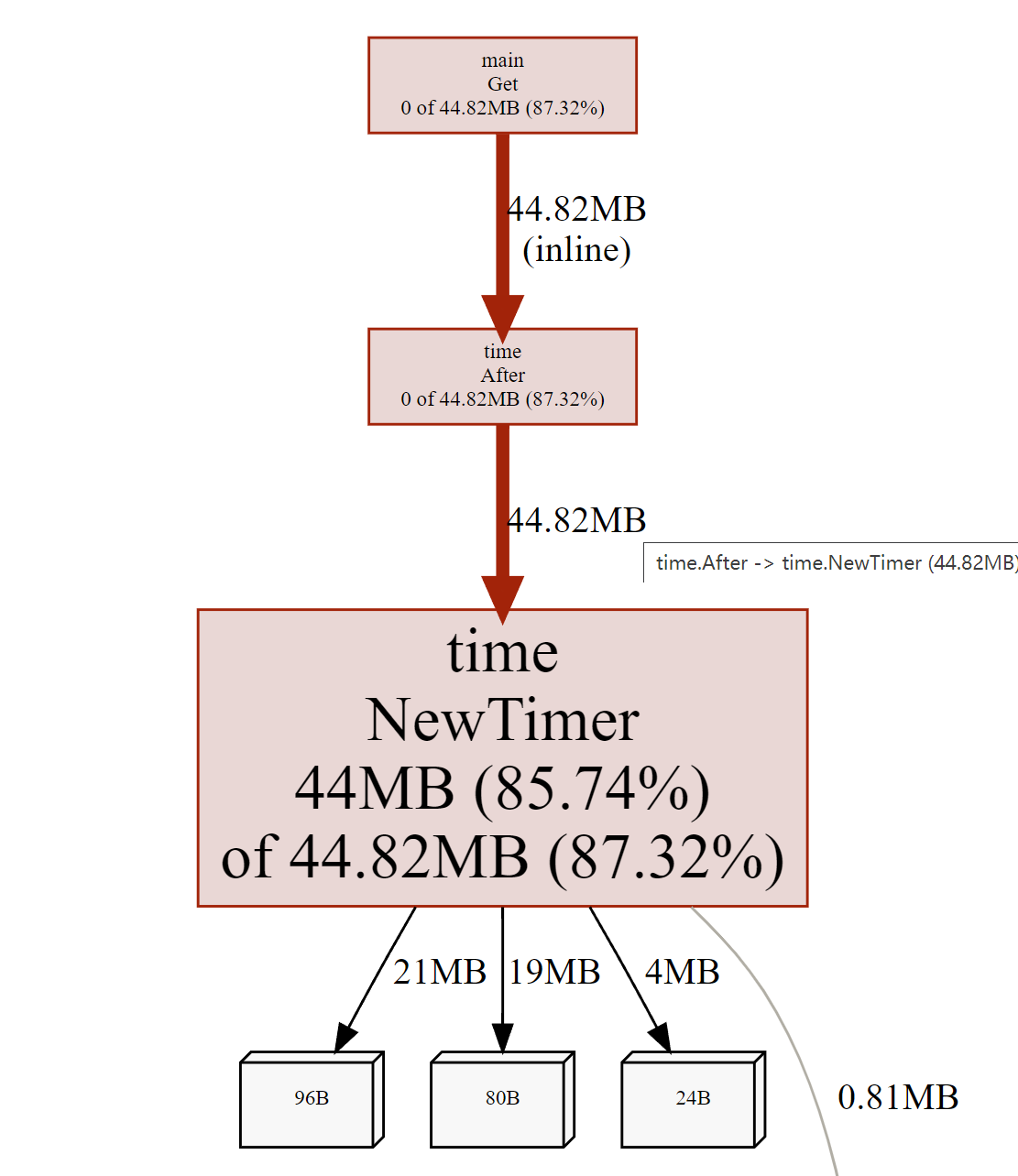I found that the memory footprint of the Timer is very high in a short while. This is because the garbage collector does not recycle the Timer until it is triggered, but each call to `time.After` inside the loop instantiates a new timer and the timer is cleared only after it is activated.

To avoid this we can use the following code:

 `````` 1 2 3 4 5 6 7 8 9 10 11 12 13 14 15 16 `````` ``````func Get() { delay := time.NewTimer(3 * time.Minute) defer delay.Stop() for { delay.Reset(3 * time.Minute) select { case v := <- chs: fmt.Printf("print:%v\n", v) case <- delay.C: fmt.Printf("time.After:%v", time.Now().Unix()) } } } ``````

## HTTP body forget Close causes leak

 `````` 1 2 3 4 5 6 7 8 9 10 11 12 13 14 15 16 17 18 `````` ``````type handler struct { client http.Client url string } func (h handler) getBody() (string, error) { resp, err := h.client.Get(h.url) if err != nil { return "", err } body, err := io.ReadAll(resp.Body) if err != nil { return "", err } return string(body), nil } ``````

The above code looks fine, but resp is of type `*http.Response`, which contains the Body `io.ReadCloser` object, which is an io class that must be closed properly, otherwise it will generate a resource leak. Generally we can do this:

 ``````1 2 3 4 5 6 `````` ``````defer func() { err := resp.Body.Close() if err != nil { log.Printf("failed to close response: %v\n", err) } }() ``````

## Cache line

Currently, there are two main memories in computers, SRAM and DRAM. main memory is implemented by DRAM, which is often referred to as memory, and there are usually three cache layers in the CPU, L1, L2, and L3, which are implemented by SRAM.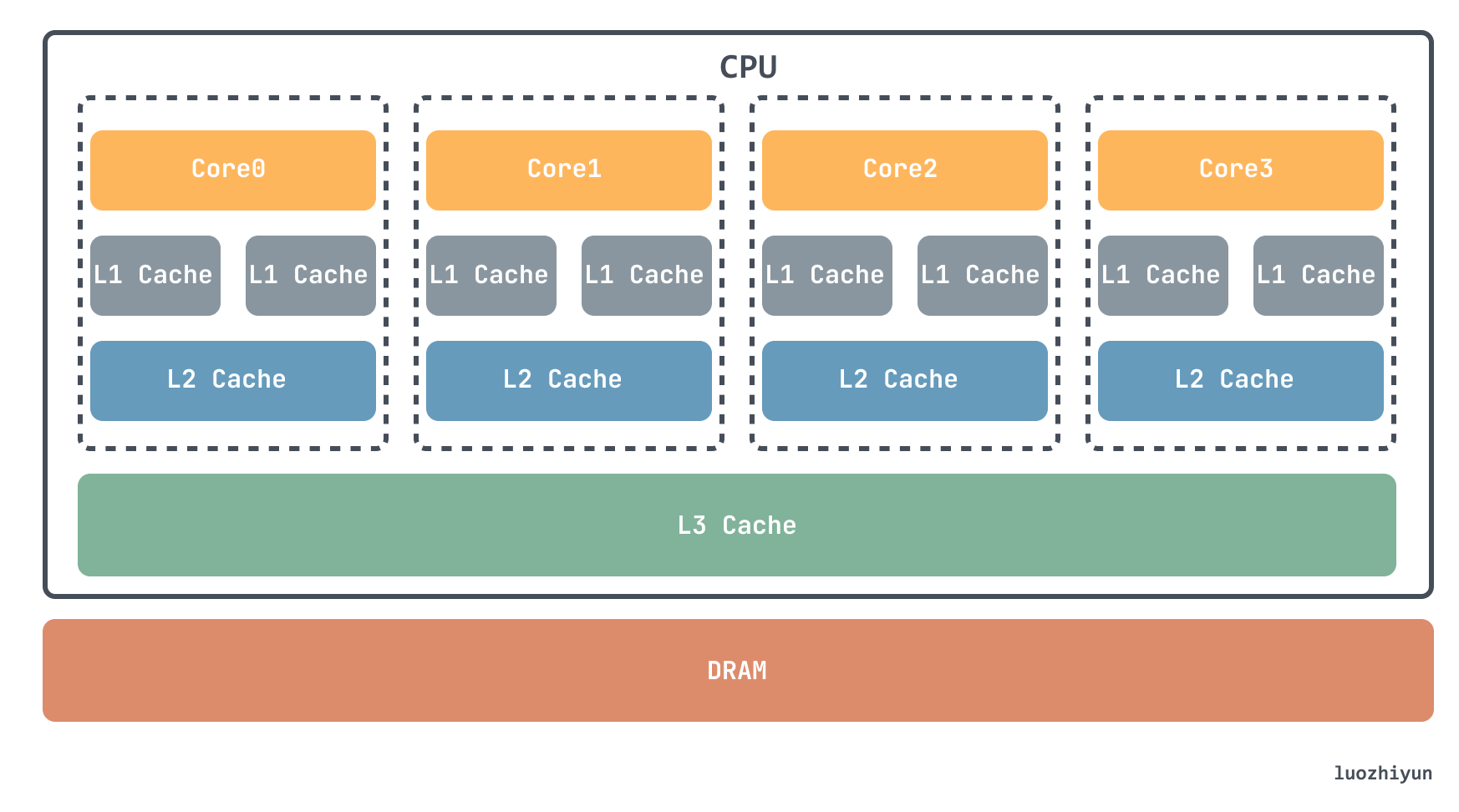When fetching units from memory to cache, it will fetch one cacheline size area of memory at a time to cache and then store it in the corresponding cacheline, so when you read a variable, you may read its adjacent variables to CPU cache as well (if they happen to be in a cacheline). Since there is a high chance that you will continue to access the adjacent variables, the CPU can use the cache to speed up memory accesses.

The cacheline size is usually 32 bit, 64 bit, 128 bit. take my computer’s 64 bit as an example.

 ``````1 2 `````` ``````cat /sys/devices/system/cpu/cpu1/cache/index0/coherency_line_size 64 ``````

We set two functions, one index plus 2 and one index plus 8.

 `````` 1 2 3 4 5 6 7 8 9 10 11 12 13 14 15 `````` ``````func sum2(s []int64) int64 { var total int64 for i := 0; i < len(s); i += 2 { total += s[i] } return total } func sum8(s []int64) int64 { var total int64 for i := 0; i < len(s); i += 8 { total += s[i] } return total } ``````

This looks sum8 processing elements than sum2 four times less, then the performance should also be about four times faster, the book said only 10% faster, but I did not measure this data, it does not matter we know that because of the existence of cacheline, and data in the L1 cache performance is very high on the line.

Then look at the slice type structure and the structure contains slice:

 `````` 1 2 3 4 5 6 7 8 9 10 11 12 `````` ``````type Foo struct { a int64 b int64 } func sumFoo(foos []Foo) int64 { var total int64 for i := 0; i < len(foos); i++ { total += foos[i].a } return total } ``````

Foo contains two fields a and b. sumFoo iterates through the Foo slice and returns all the a fields added together.

 `````` 1 2 3 4 5 6 7 8 9 10 11 12 `````` ``````type Bar struct { a []int64 b []int64 } func sumBar(bar Bar) int64 { var total int64 for i := 0; i < len(bar.a); i++ { total += bar.a[i] } return total } ``````

Bar contains two slices a, b, sumBar will return the sum of the elements of a in Bar. Let’s also test it with two benchmarks.

 `````` 1 2 3 4 5 6 7 8 9 10 11 12 13 14 15 16 17 18 19 20 21 22 `````` ``````func Benchmark_sumBar(b *testing.B) { s := Bar{ a: make([]int64, 16), b: make([]int64, 16), } b.RunParallel(func(pb *testing.PB) { for pb.Next() { sumBar(s) } }) } func Benchmark_sumFoo(b *testing.B) { s := make([]Foo, 16) b.RunParallel(func(pb *testing.PB) { for pb.Next() { sumFoo(s) } }) } ``````

Results of the test:

 ``````1 2 3 `````` ``````# go test -gcflags "-N -l" -bench . Benchmark_sumBar-16 249029368 4.855 ns/op Benchmark_sumFoo-16 238571205 5.056 ns/op ``````

sumBar will be a little faster than sumFoo. This is because for sumFoo, the entire data has to be read, while for sumBar, only the first 16 bytes have to be read into the cache line.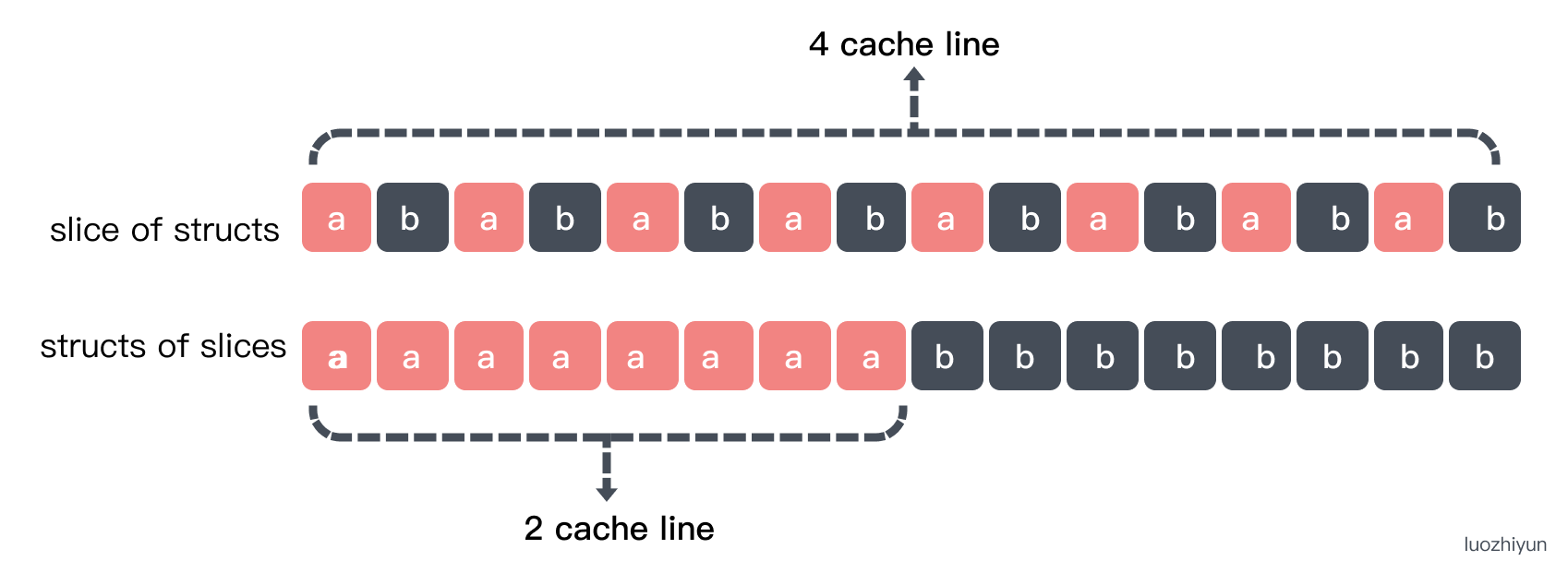## Performance issues caused by False Sharing

False sharing is a performance problem caused by repeatedly loading data into the cache due to memory cache invalidation when multiple threads read and write to the same piece of memory in parallel.

Since CPU caches are now hierarchical, and the L1 cache is exclusive to each Core, it is possible to face the problem of cache data invalidation.

If the same piece of data is loaded by multiple Cores at the same time, then it is a shared state. In the shared state, if you want to modify the data, you have to broadcast a request to all other CPU cores to invalidate the cache inside the other CPU cores before updating the data inside the current cache.

After the CPU cores become invalid, the cache can’t be used and needs to be reloaded, because the speed of different levels of cache is very different, so this actually has quite a big performance impact.

 `````` 1 2 3 4 5 6 7 8 9 10 11 12 13 14 15 16 17 18 19 20 21 22 23 24 25 26 27 28 29 30 `````` ``````type MyAtomic interface { IncreaseAllEles() } type Pad struct { a uint64 _p1 uint64 b uint64 _p2 uint64 c uint64 _p3 uint64 } func (myatomic *Pad) IncreaseAllEles() { atomic.AddUint64(&myatomic.a, 1) atomic.AddUint64(&myatomic.b, 1) atomic.AddUint64(&myatomic.c, 1) } type NoPad struct { a uint64 b uint64 c uint64 } func (myatomic *NoPad) IncreaseAllEles() { atomic.AddUint64(&myatomic.a, 1) atomic.AddUint64(&myatomic.b, 1) atomic.AddUint64(&myatomic.c, 1) } ``````

Here I define two structures, Pad and NoPad, and then we define a benchmark for multi-threaded testing.

 `````` 1 2 3 4 5 6 7 8 9 10 11 12 13 14 15 16 17 18 19 20 21 22 23 24 25 26 27 `````` ``````func testAtomicIncrease(myatomic MyAtomic) { paraNum := 1000 addTimes := 1000 var wg sync.WaitGroup wg.Add(paraNum) for i := 0; i < paraNum; i++ { go func() { for j := 0; j < addTimes; j++ { myatomic.IncreaseAllEles() } wg.Done() }() } wg.Wait() } func BenchmarkNoPad(b *testing.B) { myatomic := &NoPad{} b.ResetTimer() testAtomicIncrease(myatomic) } func BenchmarkPad(b *testing.B) { myatomic := &Pad{} b.ResetTimer() testAtomicIncrease(myatomic) } ``````

The results can be seen to be about 40% faster:

 ``````1 2 3 4 `````` ``````BenchmarkNoPad BenchmarkNoPad-10 1000000000 0.1360 ns/op BenchmarkPad BenchmarkPad-10 1000000000 0.08887 ns/op ``````

If there is no pad, the variable data will be in a cache line, so that if one thread modifies the data, the cache line of the other thread will be invalidated and need to be reloaded: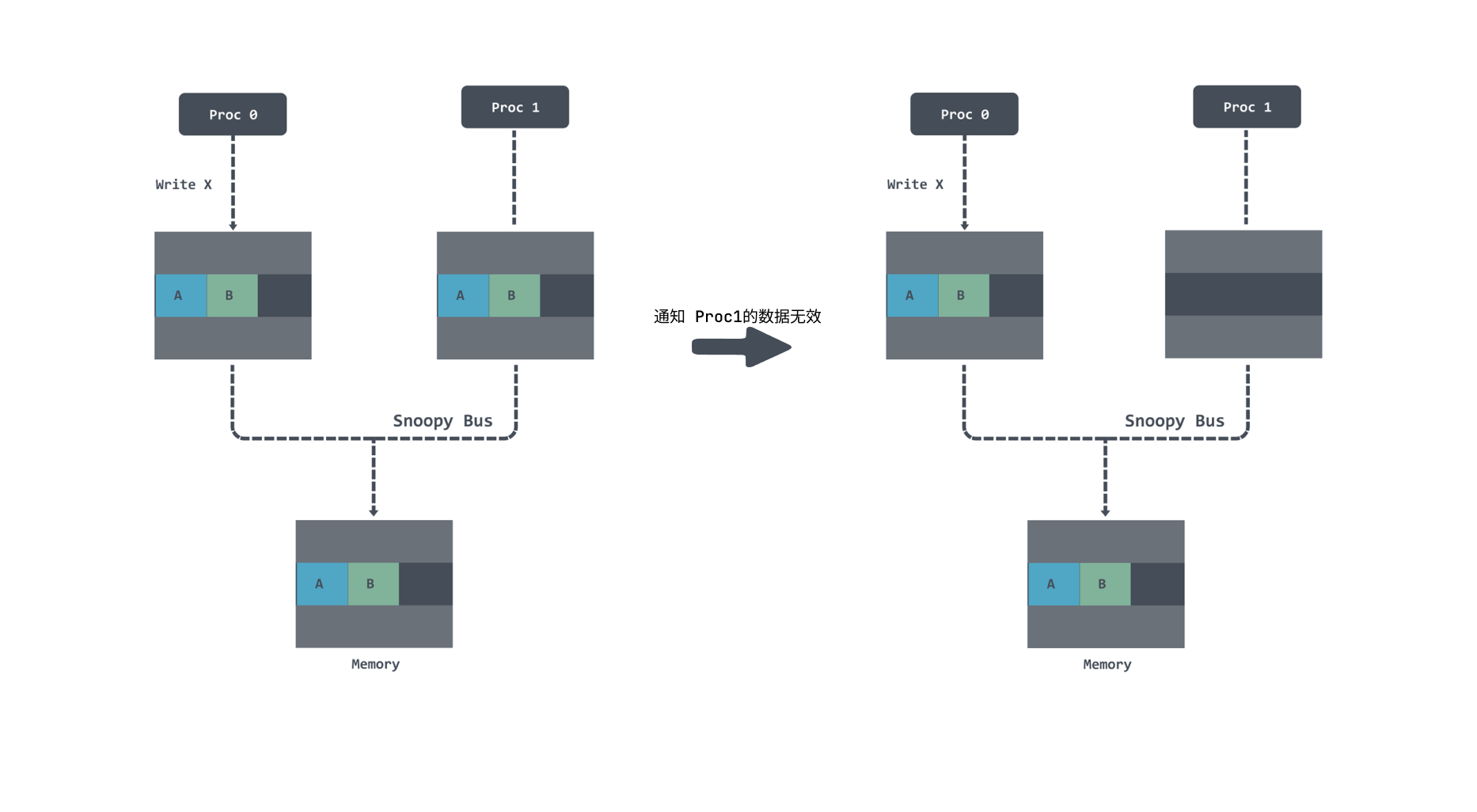After adding the padding, the data are not on the same cache line, so even if the modification is invalid, it is not necessary to reload the data on the same line.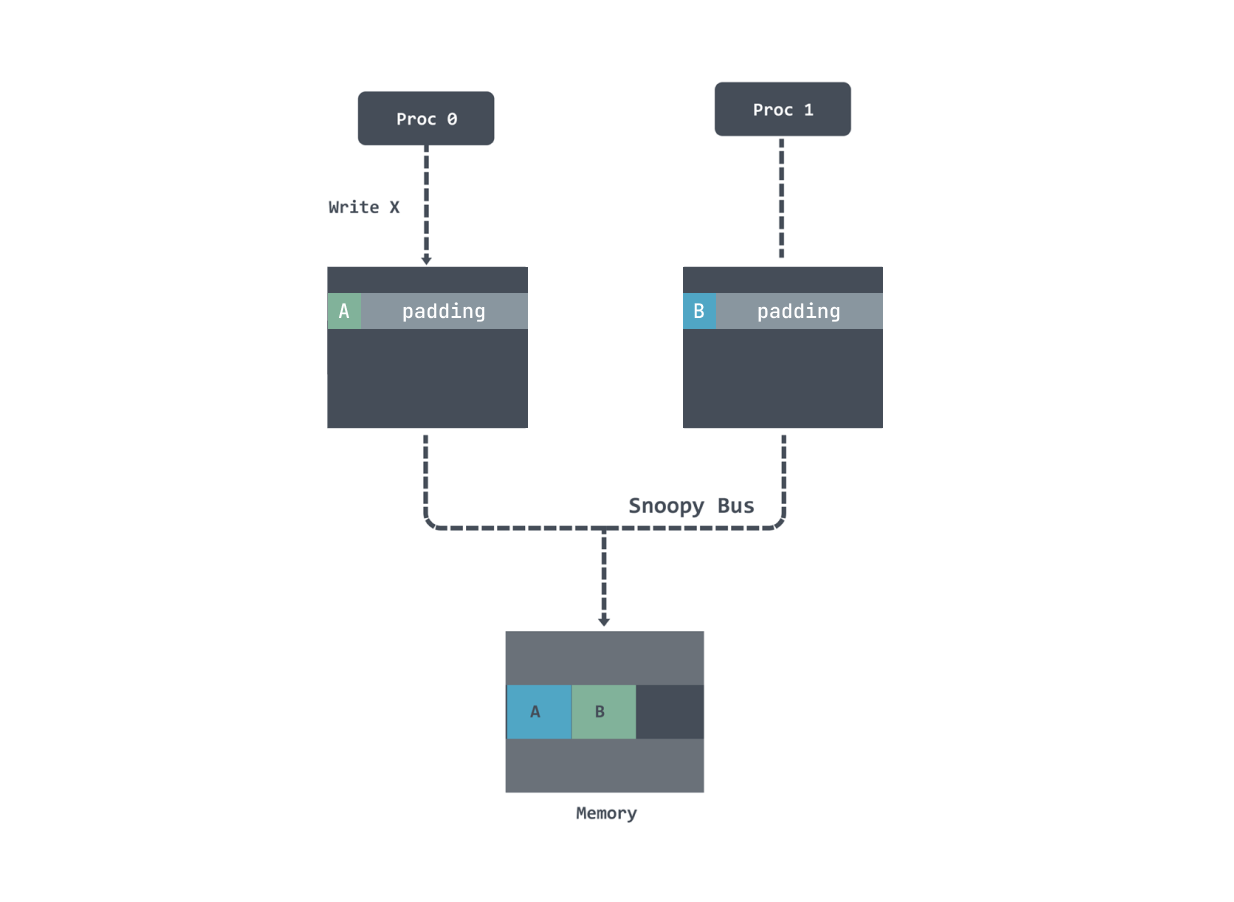## Memory Alignment

In short, nowadays CPUs access memory bytes at a time, for example, 64-bit architecture accesses 8bytes at a time, the processor can only start reading data from memory with an address that is a multiple of 8, so it requires the data to be stored with a value that is a multiple of 8 at the first address, which is called memory alignment.

For example, in the following example, because of the memory alignment, the field b in the following example can only be stored from an address that is a multiple of 8.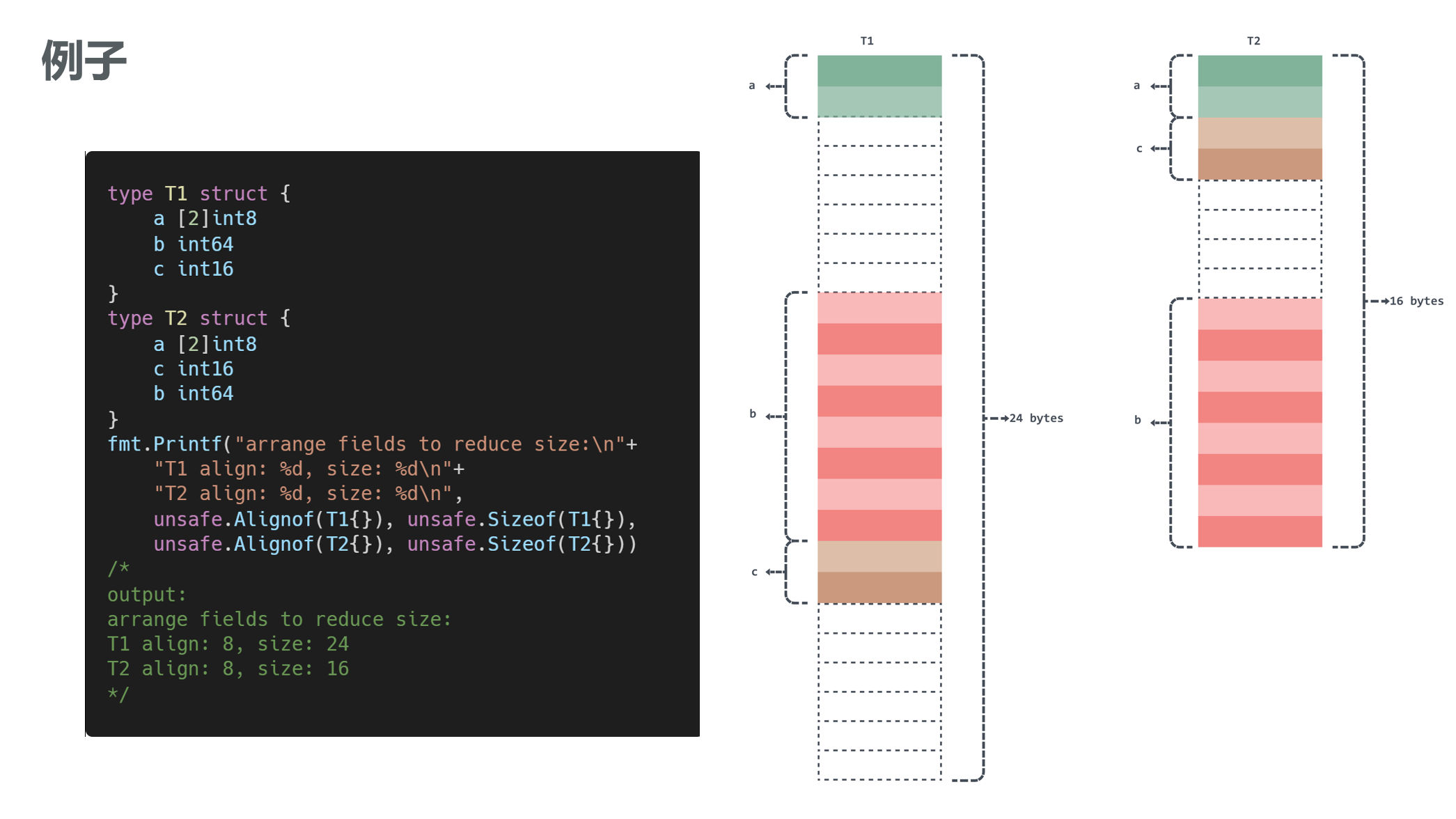In addition to this there is the issue of zero size field alignment, if a structure or array type does not contain a field or element of size greater than zero, then its size is zero. e.g. `x int8` , `empty structure struct{}` . It does not need to be aligned when it is a field, but it needs to be aligned when it is the last field of the structure. Let’s take the empty struct as an example.

 `````` 1 2 3 4 5 6 7 8 9 10 11 12 13 14 15 `````` ``````type M struct { m int64 x struct{} } type N struct { x struct{} n int64 } func main() { m := M{} n := N{} fmt.Printf("as final field size:%d\nnot as final field size:%d\n", unsafe.Sizeof(m), unsafe.Sizeof(n)) } ``````

Output:

 ``````1 2 `````` ``````as final field size:16 not as final field size:8 ``````

Of course, it is not possible to manually adjust the memory alignment, we can do so by using the tool fieldalignment:

 ``````1 2 3 4 `````` ``````\$ go install golang.org/x/tools/go/analysis/passes/fieldalignment/cmd/fieldalignment@latest \$ fieldalignment -fix .\main\my.go main\my.go:13:9: struct of size 24 could be 16 ``````

## Escape analysis

Go does escape analysis in the compiler to decide whether to put an object on the stack or on the heap, and to put objects that do not escape on the stack and those that may escape on the heap. For Go, we can see if a variable escapes by using the following directive:

 ``````1 `````` ``````go run -gcflags '-m -l' main.go ``````
• `-m` will print out the optimization strategy for escape analysis, in fact up to 4 `-m` can be used in total, but it is more informative and usually 1 is enough.
• `-l` disables function inlining, where disabling `-inlining` gives a better view of escapes and reduces interference.

### Pointer escape

An object is created in the function and a pointer to this object is returned. In this case, the function exits, but because of the pointer, the object’s memory cannot be reclaimed with the end of the function, so it can only be allocated on the heap.

 `````` 1 2 3 4 5 6 7 8 9 10 11 12 13 14 `````` ``````type Demo struct { name string } func createDemo(name string) *Demo { d := new(Demo) // Local variable d escapes to the heap d.name = name return d } func main() { demo := createDemo("demo") fmt.Println(demo) } ``````

To test it:

 ``````1 2 3 4 5 6 `````` `````` go run -gcflags '-m -l' .\main\main.go # command-line-arguments main\main.go:12:17: leaking param: name main\main.go:13:10: new(Demo) escapes to heap main\main.go:20:13: ... argument does not escape &{demo} ``````

### interface{}/any Dynamic type escapes

Because it is difficult to determine the exact type of its arguments during compilation, escapes can also occur, such as this:

 ``````1 2 3 4 5 `````` ``````func createDemo(name string) any { d := new(Demo) // Local variable d escapes to the heap d.name = name return d } ``````

### Slice length or capacity not specified escapes

If the length or capacity of a slice is known when using a partial slice, use a constant or numeric literal to define it, otherwise it will also escape:

 `````` 1 2 3 4 5 6 7 8 9 10 11 `````` ``````func main() { number := 10 s1 := make([]int, 0, number) for i := 0; i < number; i++ { s1 = append(s1, i) } s2 := make([]int, 0, 10) for i := 0; i < 10; i++ { s2 = append(s2, i) } } ``````

Output:

 ``````1 2 3 4 `````` `````` go run -gcflags '-m -l' main.go ./main.go:65:12: make([]int, 0, number) escapes to heap ./main.go:69:12: make([]int, 0, 10) does not escape ``````

### Closures

For example: `Increase()` returns a closure function that accesses an external variable n. That variable n will exist until `in` is destroyed. Obviously, the memory occupied by variable n cannot be reclaimed with the exit of function `Increase()`, so it will escape to the heap.

 `````` 1 2 3 4 5 6 7 8 9 10 11 12 13 `````` ``````func Increase() func() int { n := 0 return func() int { n++ return n } } func main() { in := Increase() fmt.Println(in()) // 1 fmt.Println(in()) // 2 } ``````

Output:

 ``````1 2 3 4 `````` `````` go run -gcflags '-m -l' main.go ./main.go:64:5: moved to heap: n ./main.go:65:12: func literal escapes to heap ``````

## Optimization of byte slice and string conversion

Directly converting `string(bytes)` or `[]byte(str)` by force will result in data replication and poor performance, so in the pursuit of extreme performance scenarios use the unsafe package approach to directly convert to improve performance.

 ``````1 2 3 4 5 6 7 8 `````` ``````// toBytes performs unholy acts to avoid allocations func toBytes(s string) []byte { return *(*[]byte)(unsafe.Pointer(&s)) } // toString performs unholy acts to avoid allocations func toString(b []byte) string { return *(*string)(unsafe.Pointer(&b)) } ``````

In Go 1.12, several methods `String`, `StringData`, `Slice` and `SliceData`, were added to do this performance conversion.

• `func Slice(ptr *ArbitraryType, len IntegerType) []ArbitraryType`: Returns a Slice, whose underlying array starts at ptr, and whose length and capacity are both len
• `func SliceData(slice []ArbitraryType) *ArbitraryType`: returns a pointer to the underlying array
• `func String(ptr *byte, len IntegerType) string`: Generate a string, the underlying array starts from ptr, length is len
• `func StringData(str string) *byte`: Returns the underlying array of strings

How these methods work can be found at: https://gfw.go101.org/article/unsafe.html.

## GOMAXPROCS in containers

Since Go 1.5, the default value of GOMAXPROCS for Go has been set to the number of CPU cores, but in a Docker or k8s container `runtime.GOMAXPROCS()` gets the number of CPU cores of the host. This can lead to `P` values being set too large, resulting in too many threads being spawned, which can increase the burden of context switching, leading to severe context switching and wasted CPU.

So you can use uber’s automaxprocs library. The general principle is to read the `CGroup` value to identify the CPU quota of the container, calculate the actual number of cores, and automatically set the number of `GOMAXPROCS` threads.

 ``````1 2 3 4 5 `````` ``````import _ "go.uber.org/automaxprocs" func main() { // Your application logic here } ``````

## Ref

• `https://go.dev/ref/mem`
• `https://colobu.com/2019/01/24/cacheline-affects-performance-in-go/`
• `https://teivah.medium.com/go-and-cpu-caches-af5d32cc5592`
• `https://geektutu.com/post/hpg-escape-analysis.html`
• `https://dablelv.github.io/go-coding-advice/%E7%AC%AC%E5%9B%9B%E7%AF%87%EF%BC%9A%E6%9C%80%E4%BD%B3%E6%80%A7%E8%83%BD/2.%E5%86%85%E5%AD%98%E7%AE%A1%E7%90%86/3.%E5%87%8F%E5%B0%91%E9%80%83%E9%80%B8%EF%BC%8C%E5%B0%86%E5%8F%98%E9%87%8F%E9%99%90%E5%88%B6%E5%9C%A8%E6%A0%88%E4%B8%8A.html`
• `https://github.com/uber-go/automaxprocs`
• `https://gfw.go101.org/article/unsafe.html`
• `https://www.luozhiyun.com/archives/797`